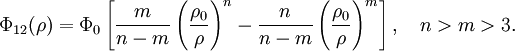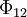# Kihara potential

(diff) ← Older revision | Latest revision (diff) | Newer revision → (diff)$\Phi_{12}(\rho)=\Phi_0 \left[\frac{m}{n-m}\left(\frac{\rho_{0}}{\rho}\right)^n-\frac{n}{n-m}\left(\frac{\rho_{0}}{\rho}\right)^m\right], ~~~ n>m>3.$
where$\Phi_{12}$ is the intermolecular pair potential, and$\rho$ is the shortest distance between molecule cores.